Bezier

# Bezier

### Overview

`bezier` implements a quadratic bezier curve

mapper. An input value between 0 and 1 goes in, and a bezier curve output comes out. The control point is controlled via X and Y parameters, presumably in the normalized range 0-1.

### Tangled Files

<<bezier.c>>=
``````#include <math.h>
#include "bezier.h"
<<static_funcdefs>>
<<funcs>>``````

<<bezier.h>>=
``````#ifndef SK_BEZIER_H
#define SK_BEZIER_H

#ifndef SKFLT
#define SKFLT float
#endif
<<funcdefs>>
#endif``````

### A bit of math

The equation for a quadratic Bezier curve is the following: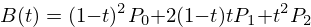Whereis a normalized time value between 0 and 1, and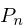refers to a 2-dimensional point with a (x,y) coordinate.

The issue with the classic equation above is that the value is derived from, allowing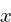to be fractional. This is problematic because the system implemented here is discrete, restricted to whole-integer values of.

The solution to this problem is to rework the equation above to solve for \$t\$ in terms of the current sample position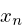. Once \$t\$ is found, it can be used to compute the result, which is the y component of the bezier curve in terms of t.

The Bezier x component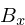can be rearranged to be a quadratic equation for, given the x bezier control points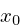,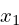, and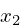, as well as the current sample position.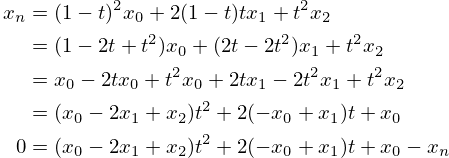This yields the following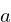,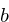, and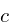quadratic equation coefficients: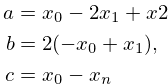Using those variables and the quadratic equation, the value ofcan be found if it is a real value.

This value is implemented in a C function called `find_t`, along with a quadratic equation `quadratic_equation`.

<<static_funcdefs>>=
``static SKFLT find_t(SKFLT x0, SKFLT x1, SKFLT x2, SKFLT x);``

<<funcs>>=
``````static SKFLT find_t(SKFLT x0, SKFLT x1, SKFLT x2, SKFLT x)
{
SKFLT a, b, c;

a = (x0 - 2.0 * x1 + x2);
b = 2.0 * (-x0 + x1);
c = x0 - x;

if (a) {
} else {
return (x - x0) / b;
}
}``````

<<static_funcdefs>>=
``static SKFLT quadratic_equation(SKFLT a, SKFLT b, SKFLT c);``

<<funcs>>=
``````static SKFLT quadratic_equation(SKFLT a, SKFLT b, SKFLT c)
{
SKFLT det; /* determinant */

det = b*b - 4*a*c;

if (det >= 0) {
return ((-b + sqrt(det))/(2.0*a));
} else {
return 0;
}
}``````

### Bezier Compute

Now, with all the mathematical derivations out of the way, a bezier curve can be computed given an x position `xpos`, and a control point at `cx` and `cy`.

<<funcdefs>>=
``SKFLT sk_bezier_tick(SKFLT xpos, SKFLT cx, SKFLT cy);``

In total the bezier takes in 3 points, with the middle point being the control point, and the end points being with the normalized ranges (0, 0) and (1, 1).

<<funcs>>=
``````SKFLT sk_bezier_tick(SKFLT xpos, SKFLT cx, SKFLT cy)
{
SKFLT x;
SKFLT y;
SKFLT t;
SKFLT val;

x = 0;
x = cx;
x = 1;

y = 0;
y = cy;
y = 1;

t = find_t(x, x, x, xpos);

val = (1.0-t)*(1.0-t)*y + 2.0*(1 - t)*t*y + t*t*y;
return val;
}``````